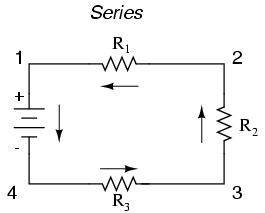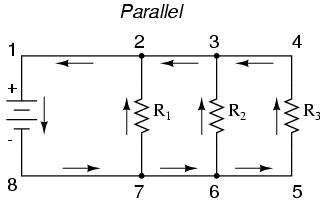Home   |  Schematics |  Products |  Tutorials  |  Datasheets  |  Robotics   |   Download    |   Link Exchange

 » Experiment » Calculator/Converters » Radio » Newsletter » Associations and Societies » Component Manufacturers

 Electronics Symentics A B C D E F G H I J K L M N O P Q R S T U V W X Y Z

 Series Parallel Combination Circuits

 With simple series circuits, all components are connected end-to-end to form only one path for electrons to flow through the circuit:With simple parallel circuits, all components are connected between the same two sets of electrically common points, creating multiple paths for electrons to flow from one end of the battery to the other:With each of these two basic circuit configurations, we have specific sets of rules describing voltage, current, and resistance relationships. Series Circuits: Voltage drops add to equal total voltage. All components share the same (equal) current. Resistances add to equal total resistance. Parallel Circuits: All components share the same (equal) voltage. Branch currents add to equal total current. Resistances diminish to equal total resistance.